# Linear Regression Explained

Linear Regression is one of the most fundamental algorithms in Machine Learning you will ever encounter. Linear Regression involves fitting a linear function through the data which can be used to predict a continuous linear value like prices, stocks, houses, etc. A continuous value is anything that can be any real number. Regression involves predicting continuous real values and classification involves predicting classes. But this article will only cover regression.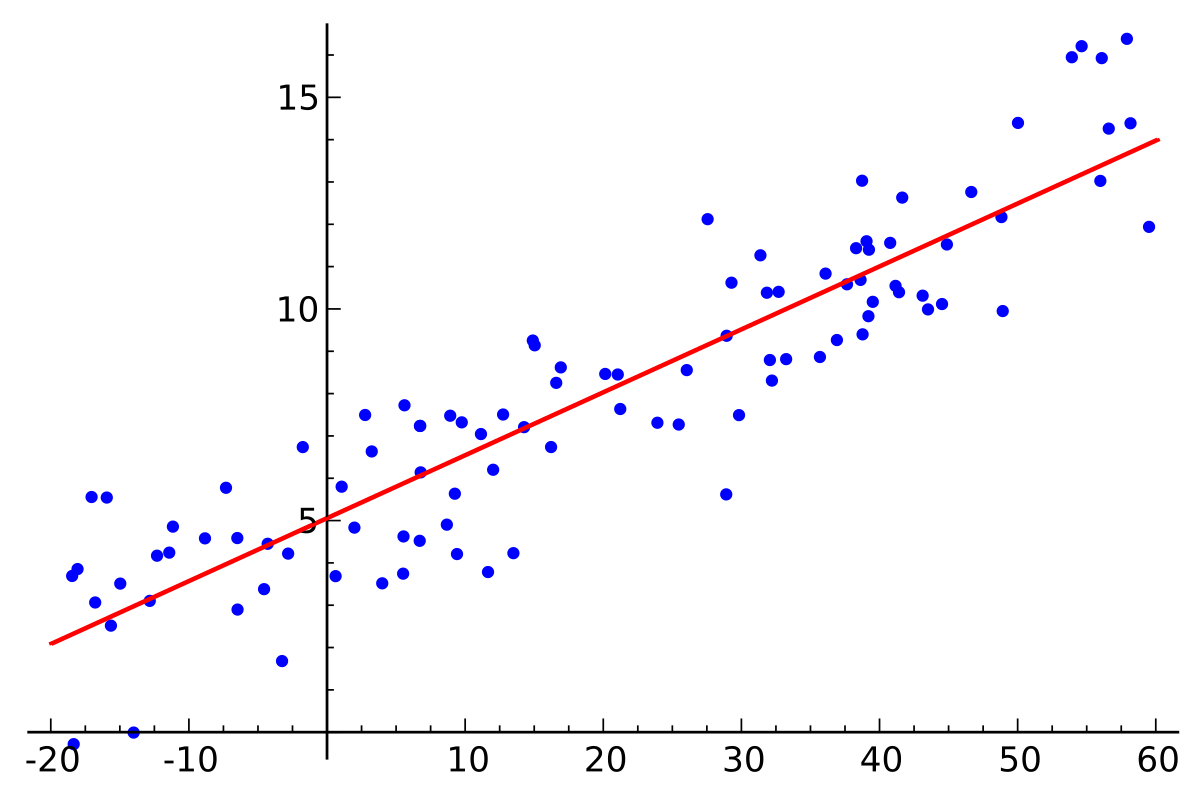This shows linear regression where the blue points are the training examples and the red line is the line of best-fit Image by https://en.wikipedia.org/wiki/Linear_regression

Note: This article will use university mathematics so a strong grasp on multivariable calculus (especially partial derivatives) and linear algebra is recommended.

This article will teach you how single variable and multivariable Linear Regression works. Let’s first look at a classic Linear Regression problem-

This is a classic Linear Regression problem which involves predicting house prices using its features like its area, bedrooms, etc. We are covering single variable linear regression so we will only use one feature to predict the price which for our example is the number of bedrooms. Let’s go over some machine learning terminology.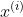This represents the input (no. of bedrooms) of the i-th training example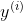This represents the output (the price of the house) of the i-th training example

The computer will try to find a linear function which fits the data best. This linear function is called the hypothesis.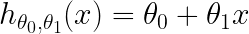This is our hypothesis, with two parameters theta 0 and theta 1

The computer will try to adjust the two parameters, theta 0 and theta 1 such that the error is minimized. The question is, how would you find the error and how would you minimize it? This is where the cost function comes in.

The cost function which is also sometimes called the loss function is the function which measures how the computer performs. The most common cost function for linear regression is mean squared error.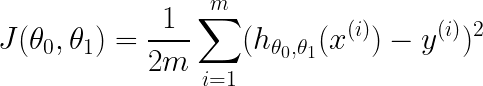This is the mean squared error function where m is the number of training examples and theta 0 and theta 1 are the two parameters of the cost function and the hypothesis

The cost function makes the job much easier because now the task is reduced to just minimizing the cost function. One of the most commonly used algorithms for optimization is Gradient Descent.

Gradient Descent is like climbing down a hill until you find a local minimum. If you have a strong grasp of multivariable calculus, then you will know that the gradient is the line of steepest ascent so it follows from that the negative of the gradient is the line of steepest descent. So first you initialize theta 0 and theta 1 randomly then you take steps in the direction of the negative gradient. The size of the steps you take is dependent on the learning rate you choose. If you choose a really big learning rate you might skip the local minimum and if you choose a really small learning rate you will find the local minimum but the training time for the machine learning model will be really long. You will need to experiment with different learning rates to find the perfect learning rate value for your task.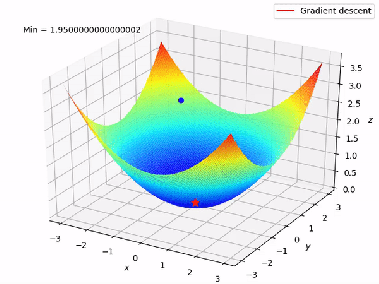This shows how the gradient descent algorithm finding the local minimum in the graph of the cost function Image by- https://suniljangirblog.wordpress.com/2018/12/03/the-outline-of-gradient-descent/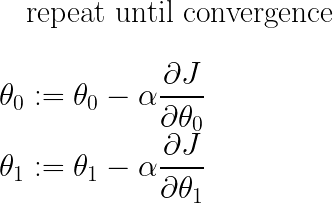These equations show how to adjust the two parameters where := is the assignment operator, and alpha is the learning rate

Now we will look at multivariable regression. In multivariable linear regression, we have multiple features to consider unlike the previous example where we only considered the number of bedrooms. For example, considering the area of the lawn, area of the house, etc also. Multivariable linear regression is very similar to its single variable counterpart with only a few changes but the main idea stays the same. There is some changes in the terminology in multivariable linear regression.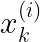This represent the k-th feature in the i-th training example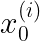We define this feature to be equal to 1 for notation purposes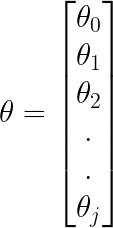Theta is now a vector of parameters where j is the number of features for each training examples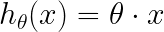Our hypothesis has now a single parameter which is a vector now and x is also a vector. The hypothesis is the dot product of the two vectors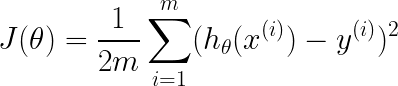Our cost function now also takes a single parameter theta, which is now a vector

Now we can apply gradient descent to the cost function. Gradient Descent nearly stays the same with only a slight change.